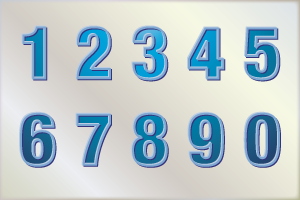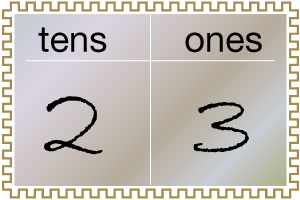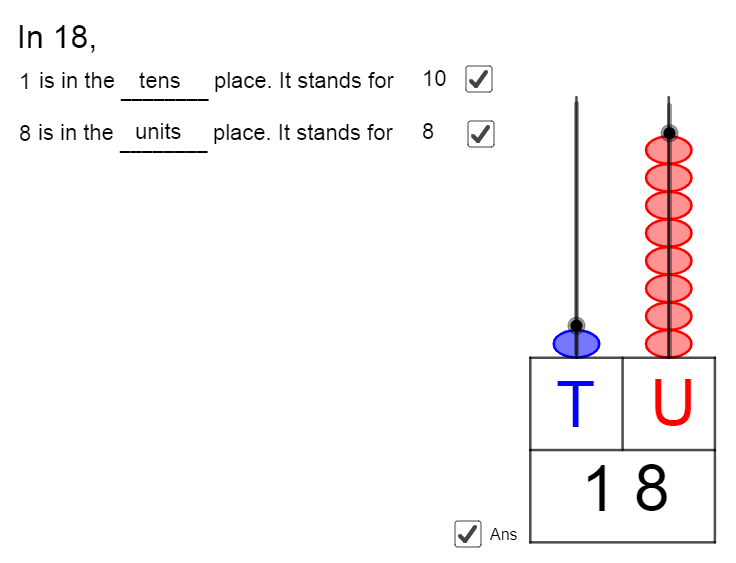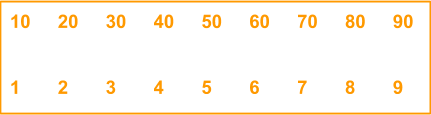# Numbers up to 2-Digits

Go back to  'Numbers and Place values'

## Introduction to Numbers up to 2-Digits

We begin counting numbers on the fingers of our hands at a very young age, and then go on to using supercomputers to crunch numbers which are very large. Whatever be the count of digits of a number, the correct placement of each digits is most important. Today we will discuss $$2$$-digit numbers, but before that, let us familiarize ourselves with single digit numbers. There are $$10$$ single digit numbers $$-0, 1, 2, 3, 4, 5, 6, 7, 8,$$ and $$9.$$ All the double digit or $$2$$-digit numbers we will discuss are combinations of any two of nine of these ten numbers. Yes, $$0$$ is not always counted, and we will come to that later.## The Big Idea: Numbers up to 2-Digits

### A simple idea: The Place Value of Numbers

Every number which has more than $$1$$ digit has to have the different digits described by their place values. The place values we use are units, tens, hundreds, thousands, ten thousands and so on. You will notice that each of the consecutive place values is ten times the previous one. When we limit ourselves to $$2$$-digit numbers, therefore, there are only two place values in play – the units place and the tens place.Let us say you buy ice cream from a shop, and you have $$5$$ friends coming over for lunch. Including your parents, you need $$5$$ $$+$$ You $$+$$ Parents = $$8$$ ice creams. You can go to the shop and buy $$8$$ units of ice creams.

If you invite your whole class home when you are ready to leave school, you have $$33$$ friends $$+$$ you $$+$$ your parents = $$36$$ ice creams. So, you will take $$3$$ tubs to give you the equivalent of $$30$$ ice creams, and take $$6$$ ice cream cups, making it $$36$$ ice creams in all.

This is exactly what is done when we create $$2$$-digit numbers.### Assigning Place Values

So, every set of ten units becomes a number with a place value of ten, just like the shopkeeper made 1 tub by combining $$10$$ ice cream cups. Let us look at $$3$$ sets of numbers to understand the conversion of units to a combination of units and tens:

\begin{align} &{\text{33 units: 3 Tens + 3 Units}}\\ &{\text{8 units: 1 Ten + 8 Units}}\\ &{\text{27 units: 2 Tens + 3 Units}} \end{align}

I am sure you have been able to make out that only the first one is correct. The second one has the tens place wrong, and the third one has the units place wrong. This is what the right combinations would be for both sets:

\begin{align} { 3 \text { Tens } + 3 \text { Units } \\= ( 3 \times 10 ) + ( 3 \times 1 ) \\= 30 + 3 = 33 } \\ { 1 \text { Ten } + 8 \text { Units } \\= ( 1 \times 10 ) + ( 8 \times 1 ) \\= 10 + 8 = 18 } \\ { \text { 2 Tens } + 3 \text { Units }\\ = ( 2 \times 10 ) + ( 3 \times 1 ) \\= 20 + 3 = 23 } \end{align}

\begin{align}& 33 \text { units: } \\&( 3 \times 10 ) + ( 3 \times 1 ) \\&= 30 + 3 = 33 \\ &8 \text { units: }\\ &( 0 \times 10 ) + ( 8 \times 1 ) \\&= 0 + 8 = 8 \\ & 27 \text { units: }\\& ( 2 \times 10 ) + ( 7 \times 1 )\\& = 20 + 7 = 27 \end{align}### Some Common $$2$$ – digit numbers

Let us see if you know the answers to the following $$5$$ questions:

1. How many numbers does a dozen have?
2. What is considered an unlucky number?
3. What is a score?
4. How many yards long is a cricket pitch?
5. What number is a quarter century?

The answers to the above questions in order are: $$12, 13, 20, 22$$ and $$25.$$ All these five numbers are $$2$$-digit numbers.

## How is it important?

### The Significance (or otherwise) of Zero in $$2$$-digit numbers

Since we already know that a $$2$$-digit number can be formed by combinations of two of the $$10$$ digits from $$0$$ to $$10.$$ But what about those numbers which have $$0$$ in the ten’s place value? Let us consider the number that would be formed by zero in ten’s place and five in unit’s place.

The number is \begin{align} ( 0 \times 10 ) + ( 5 \times 1 ) = 0 + 5 = 5 \end{align}

So it actually becomes a single digit number. But that is not the case when the zero is in the unit’s place. Let us try exchanging the numbers this time, and take five in ten’s place and zero in unit’s place.

The number is \begin{align} ( 5 \times 10 ) + ( 0 \times 1 ) = 50 + 0 = 50 \end{align}

So when it comes to zero, there are nine options which have zero in ten’s place when the number formed is a single digit number, irrespective of which number is in the unit’s place.

## Tips and Tricks

• Tip: Help children see numbers as flexible quantities. A useful tip is to ask students to break numbers in many different ways. Eg. 15 is 10 and 5 more. At the same time, 15 is also 14 and 1 more. It is also 11 and 4 more. Play this game often with different numbers. Start with small numbers like 15 and 20 and then move to larger numbers like 32 and 46.
• Tip: Help children become very comfortable with skip counting by 10. They should be able to skip count by 10 from any number. Start with the series 10, 20, 30,... but then build comfort by getting students to skip count by 10 from any other starting point. Eg. from 23: 23, 33, 43, 53,...
• Tip: List numbers in 10s in one row and one in the second row. Show the patterns of how numbers from 11 to 99 can be constructed by combining the tens and ones.### Common mistakes or misconceptions

• Children can often recite all numbers till 99 (or 100) but may make mistakes when asked to find a larger number between two numbers.
Students sometimes recite numbers from 1 to 100 like they have memorised the letters of the alphabet. If this happens, then finding the greater number of 14 and 15 is as puzzling as finding the greater letter between C and D. Children must realise that unlike letters, numbers have a relationship with each other. 15 is exactly one more than 14. For this use number lines, colour counters to associate the sound with the quantity.

• Children tend to make mistakes working with numbers when the tens place value changes. E.g. when going from 19 to 20, 29 to 30, 39 to 40, etc.
Often 2-digit numbers are introduced without reference to place values. While this makes it easier to memorise numbers, it may not be the best strategy as children don’t see numbers as constructed from a common set of digits - 0 to 9. Best to use an abacus to model numbers and use Base-10 blocks to help them visualise numbers.

• Children make errors when spelling numbers. Especially from eleven to nineteen.
There is no substitute to practise. Children must practise writing number names. Generally, with a little bit of deliberate and targetted practice, this error can be fixed easily.